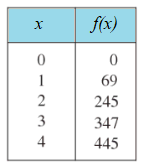# Rate of Change: Instantaneous, Average

Rate of change” is exactly what is sounds like: it’s a measure (a rate) of how things are changing. For example:

• How fast a car accelerates (or decelerates),
• The rate at which a balloon fills with hot air,
• How fast or slow a particle moves in the Large Hadron Collider.

Basically, if something is moving (and that includes getting bigger or smaller), you can study the rate at which it’s moving (or not moving).

## Average Rate of Change

The average rate of change of a function gives you the “big picture” of an object’s movement. It’s a ballpark average that gives you a good idea of how long its going to take to get from a to b, even if the object you’re studying doesn’t always move along at a steady rate. Cars accelerate over dips and hills, spaceships fly through different air densities, balloons fill at different rates depending on their volume, and so on.

The “average” of a function gives you a good idea about how fast or how slow something is happening. It’s found with the following formula:

If you’ve worked with the slope formula, this should look fairly familiar. The two formulas are practically identical, except for the notation (the slope formula is m = change in y / change in x). The main difference is that the slope formula is really only used for straight line graphs (a.k.a. linear functions). The average rate of change formula is also used for curves.

## Examples

When calculating the average rate of change, you might be given a graph, a formula, or a table. Watch the video for three short examples (one of each type):

Example Question 1: Use the following table to find the average rate of change between x = 0 and x = 1.Solution:

Step 1: Place the x-values into the formula:Step 2: Place the y-values into the formula:
Note: “f(x)” is notation for the function output, which is really just another name for the y-value.Step 3: Solve:That’s it!

## Instantaneous Rate of Change

The instantaneous rate of change is another name for the derivative. While the average rate of change gives you a bird’s eye view, the instantaneous rate of change gives you a snapshot at a precise moment. For example, how fast is a car accelerating at exactly 10 seconds after starting?

A general formula for the derivative is given in terms of limits:## Instantaneous Rate of Change Example

Example question: Find the instantaneous rate of change (the derivative) at x = 3 for f(x) = x2.

Step 1: Insert the given value (x = 3) into the formula, everywhere there’s an “a”:Step 2: Figure out your function values and place those into the formula. The function is given to you in the question: for this example, it’s x2. That means you’re going to square your function values:

• f(3), shorthand notation for “the y value at x = 3”, is going to be 32.
• f(3 + h) is (3 + h)2.Step 3: Solve the formula. This is where strong algebra skills will come in handy. At this point, the calculus is done—all you have to do is solve. The 32 at the end is easy (= 9) but the (3 + h)2 needs you to multiply out (3 + h)(3 + h). If you’ve done the algebra correctly, you’ll end up with:The +9 and -9 cancel out, leaving:Dividing by h leaves (6 + h).

We’re looking for a limit as h→0, so that leaves just 6.

The instantaneous rate of change is just 6.

That’s it!

You might find all that algebra a little challenging. The good news is, once you learn the derivative rules (shortcuts for finding them!), life becomes a lot simpler. You won’t have to work the limit formula any more, and the algebra becomes a lot less labor-intensive.

## References

Stewart et. al. (2010). College Algebra: Concepts and Contexts. Cengage Learning.

CITE THIS AS:
Stephanie Glen. "Rate of Change: Instantaneous, Average" From StatisticsHowTo.com: Elementary Statistics for the rest of us! https://www.statisticshowto.com/calculus-definitions/rate-of-change-instantaneous-average/